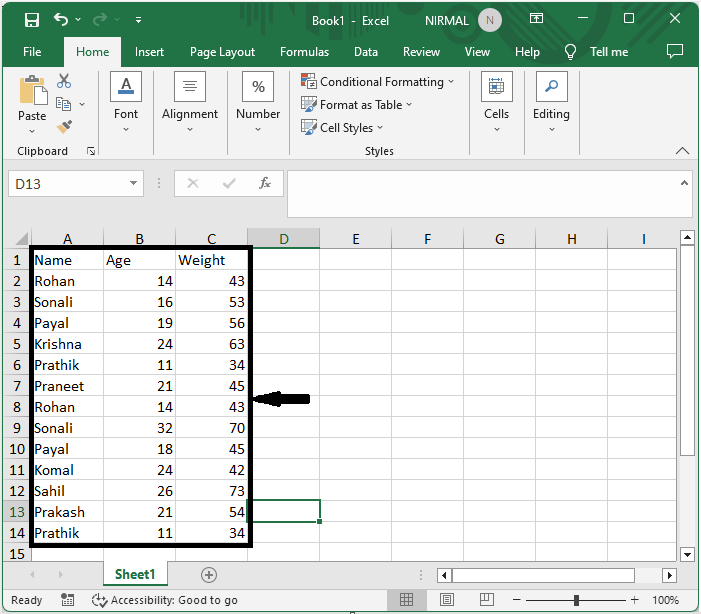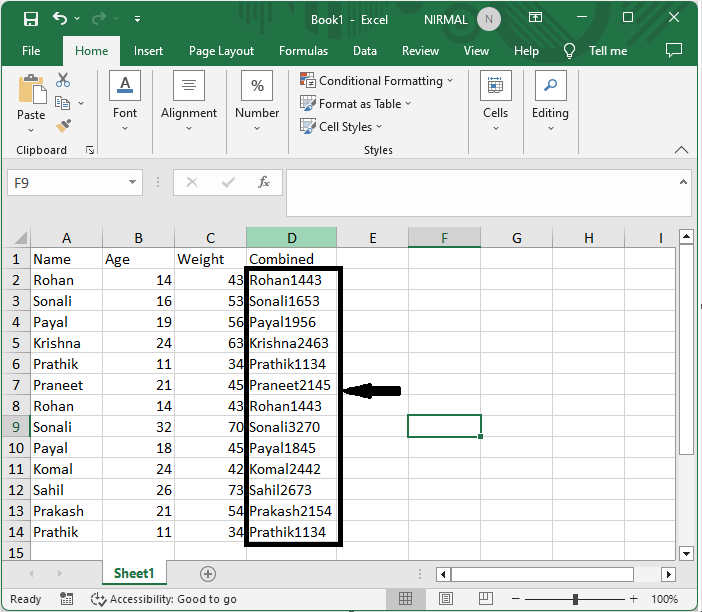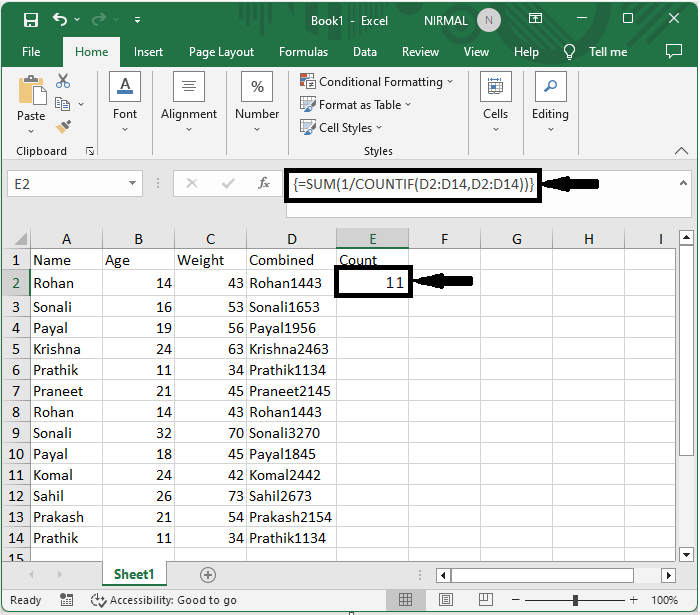# How to Count the Number of Unique Rows (Combinations of Multiple Columns) in Excel?

Excel is a strong programme that is frequently used for managing and analysing data. Finding the number of unique rows based on combinations of several columns is a typical problem in data analysis. This enables us to locate and examine particular data sets inside a dataset. When working with huge datasets or trying to find unique records in a dataset containing duplicate entries, counting the number of unique rows is especially helpful. Several built-in functions and methods in Excel can be used to efficiently complete this operation.

This tutorial will take you step-by-step through the procedure, enabling you to efficiently analyse your data and get insightful knowledge whether you are a novice or seasoned Excel user. So, let's get started and discover how to determine how many distinct rows there are in Excel!

## Count the Number of Unique Rows (Combinations of Multiple Columns)

Here we will first combine the columns and then count the number of columns needed to complete the task. So let us see a simple process to learn how you can count the number of unique rows (combinations of multiple columns) in Excel.

### Step 1

Consider an Excel sheet where the data in the sheet is similar to the below image.First, click on an empty cell, in this case, cell D2, and enter the formula as =A2&B2&C2 and click enter to get the first value. Then drag down using the autofill handle.

Empty cell > Formula > Enter.### Step 2

Then again, click on an empty cell and enter the formula as

=SUM(1/COUNTIF(D2:D14,D2:D14)) and click enter to complete the task.

Empty cell > Formula > Enter.This is how you can count the number of unique records in Excel.

## Conclusion

In this tutorial, we have used a simple example to demonstrate how you can count the number of unique rows (combinations of multiple columns) in Excel to highlight a particular set of data.

Updated on: 22-Aug-2023

394 Views